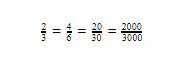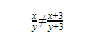GRE Math for People Who Hate Math: RatiosYou can attend the first session of any of our online or in-person GRE courses absolutely free. Crazy, right? Check out our upcoming courses here.

I recently had a great conversation about ratios with one of our MPrep GRE classes. It’s a tiny class, and only two students were there that day (hey guys!). When I shared a tricky ratio problem with them, both students had totally different, but equally reasonable, reactions to it. Here’s the problem:

The ratio of boys to girls in a class is 2 to 3. If 6 boys and 6 girls join the class, what is the new ratio of boys to girls?

The truth is, this is a trick question. But before I reveal why, I’d like to share the two different ways that my students approached it.

One student reasoned about it like this:

The ratio is initially 2 boys to 3 girls. When 6 boys and 6 girls join the class, you should end up with a ratio of 2+6=8 boys to 3+6=9 girls. The answer is 8 to 9.

The other student reasoned about it like this:

A ratio is a relationship between two quantities. If we add the same number of boys as girls, the relationship between boys and girls shouldn’t change. The answer is 2 to 3.

Both of those are pretty logical. However, neither 8 to 9, nor 2 to 3, is the right answer. Let’s understand why.

The fundamental problem with ratios is that they don’t give you very much information. When the problem tells you that the ratio of boys to girls is 2 to 3, it’s leaving a lot of possibilities open. You could have exactly 2 boys and exactly 3 girls. You could have 20 boys and 30 girls. You could even have 200 boys and 300 girls. As long as there are 2 boys for every 3 girls, there could be any number of students in the class.

However, in order to calculate ratios on the GRE, you usually need to know exact numbers. In the problem above, you’re asked to find the new ratio between boys and girls. That actually depends on exactly how many boys and girls there are.

If there were 2 boys and 3 girls to start out with, the new number of boys is 8, and the new number of girls is 9. The new ratio is 8 to 9. If there were 20 boys and 30 girls to start out with, the new number of boys is 26, and the new number of girls is 36. The new ratio is 26 to 36. Simplify this by dividing both parts by 2: this ratio is 13 to 18. If there were 200 boys and 300 girls, the new ratio will be 206 to 306, or 103 to 153. All three of those ratios are different, and all three of them are correct.

So, what’s the right answer to the question? “It depends on how many boys and girls there are.” Since you don’t know how many boys and girls there are, you can’t figure out the right answer.

Let’s look at the two ways of reasoning about ratios from earlier in the article. The first student assumed that the ratio would give her the exact numbers. Remember that any time a problem gives you a ratio, there are many different numbers that could work. This is a good opportunity to try different sets of numbers, to check whether the answer changes.

The second student made a more subtle mistake. If we had multiplied the number of boys and the number of girls by 6, the relationship would have stayed the same. The ratio would have been 12 to 18, which simplifies back down to 2 to 3. However, adding the same number of boys and girls doesn’t have that effect.

You can always multiply or divide both parts of a ratio by the same value (just like you can with a fraction).

2 to 3 = 4 to 6 = 20 to 30 = 2000 to 3000You can’t add the same value to both parts of a ratio. Doing that changes the value of the ratio.

2 to 3 ≠ 8 to 9

x to y ≠ (x + 3) to (y + 3)

Fractions work the same way!That’s why you can’t “cancel” the two matching 3s in the expression above, by the way! It changes the value of the fraction.

In short, when you’re dealing with ratios, be cautious and test numbers. A ratio doesn’t give you much information about the actual numbers, so it pays to avoid assumptions. It can also help to remember that ratios are the same as fractions. If you can do something to a fraction—like, for instance, multiplying the top and bottom by the same value—you can also do it to a ratio. If you can’t—for instance, you can’t add two fractions together without first finding a common denominator—you also can’t do it with ratios. ?

See that “SUBSCRIBE” button in the top right corner? Click on it to receive all our GRE blog updates straight to your inbox!

Chelsey Cooleyis a Manhattan Prep instructor based in Seattle, Washington. Chelsey always followed her heart when it came to her education. Luckily, her heart led her straight to the perfect background for GMAT and GRE teaching: she has undergraduate degrees in mathematics and history, a master’s degree in linguistics, a 790 on the GMAT, and a perfect 170Q/170V on the GRE. Check out Chelsey’s upcoming GRE prep offerings here.